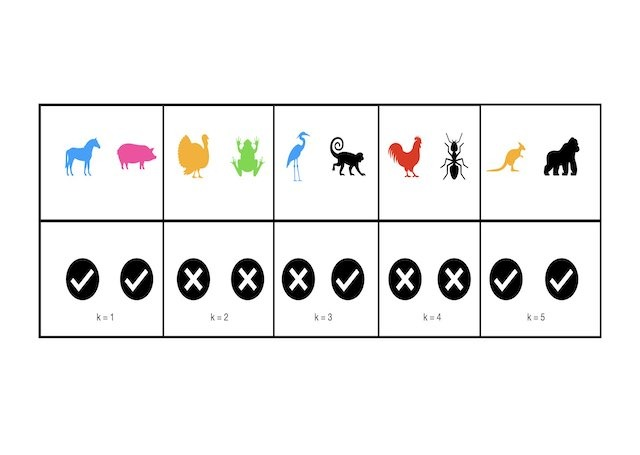# Ensembles

This category groups articles on the topic of ensemble learning methods. Each post focuses on a specific ensemble learning algorithm. The emphasis here is on understanding these models at a technical level. Here you will learn to build ensemble models in Python from scratch.## Implement Gradient Boosting Regression in Python from Scratch

Implement Gradient Boosting Regression in Python from Scratch In this post, we will implement the Gradient Boosting Regression algorithm in Python. This is a powerful supervised machine learning model, and popularly used for prediction tasks. To gain a deep insight into how this algorithm works, the model will be built up from scratch, and subsequently verified against the …## Understanding the Gradient Boosting Regressor Algorithm

Understanding the Gradient Boosting Regressor Algorithm In this post, we will cover the Gradient Boosting Regressor algorithm: the motivation, foundational assumptions, and derivation of this modelling approach. Gradient boosters are powerful supervised algorithms, and popularly used for predictive tasks. Motivation: Why Gradient Boosting Regressors? The Gradient Boosting Regressor is another variant of the boosting ensemble technique that …## A Complete Introduction to Cross Validation in Machine Learning

A Complete Introduction to Cross Validation in Machine Learning This post will discuss various Cross Validation techniques. Cross Validation is a testing methodology used to quantify how well a predictive machine learning model performs. Simple illustrative examples will be used, along with coding examples in Python. What is Cross Validation? A natural question to ask, when …## Understanding the Adaboost Regression Algorithm

Understanding the Adaboost Regression Algorithm In this post, we will describe the Adaboost regression algorithm. We will start with the basic assumptions and mathematical foundations of this algorithm, and work straight through to an implementation in Python from scratch. Motivation: What is a Adaboost Regressor? In this post, we will describe the Adaboost regression algorithm. We will …## Understanding the Adaboost Classification Algorithm

Understanding the Adaboost Classification Algorithm In this post, we will describe the Adaboost classification algorithm. We will start with the basic assumptions and mathematical foundations of this algorithm, and work straight through to an implementation in Python from scratch. Motivation: What is a Adaboost Classifier? In this post, we will describe the Adaboost classification algorithm. We will …## Introduction to Simple Boosting Classification in Python

Introduction to Simple Boosting Classification in Python This post will consist of a simple introduction to boosting classification in Python. In my previous article, I introduced boosting with a basic regression algorithm. Here, we will adapt that procedure to handle classification problems. Motivation for Boosting Classification Boosting is a popular ensemble technique, and forms the …## Introduction to Simple Boosting Regression in Python

Introduction to Simple Boosting Regression in Python This post will consist of an introduction to simple boosting regression in Python. Boosting is a popular ensemble technique, and forms the basis to many of the most effective machine learning algorithms used in industry. For example, the XGBoost package routinely produces superior results in competitions and practical applications. …## Build a Random Forest in Python from Scratch

Build a Random Forest in Python from Scratch For this post, we will Build a Random Forest in Python from scratch. I will include examples in classification and regression. Motivation to Build a Random Forest Model Bagging ensembles are an approach to reduce variance, and thereby increase model performance. In this algorithm, multiple weak learner models produce predictions …## Build a Bagging Classifier in Python from Scratch

Build a Bagging Classifier in Python from Scratch In this article, we will build a bagging classifier in Python from the ground-up. Our custom implementation will then be tested for expected behaviour. Through this exercise it is hoped that you will gain a deep intuition for how bagging works. Motivation to Build a Bagging Classifier …